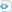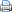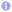C++

# C++11/14 for scientific computing IV

## Random numbers

C++98 inherited from C the standard functions rand and srand and the macro RAND_MAX for generating pseudo-random numbers. These functions suffer several problems. For example, it has been never specified which algorithm is used in rand to produce pseudo-random numbers. Thus, in C and in C++98 the outcome of a Monte Carlo simulation depends on the employed implementation of the standard library if rand is used. Furthermore, in C++98 it is hard to generate random numbers from some non-uniform distribution.

Therefore, in C++11 a portable random number generator facility has been introduced. All classes related to random number generation are declared in the header random. The random number generator facility consists of engines and distributions. Engines produce streams of pseudo random bit patterns and may implement various algorithms for random number generation, e.g., linear congruences or the Mersenne Twister. Distributions consume an engine’s bits to generate random numbers, which are distributed according to a specific distribution, e.g., uniform, exponential or Gaussian. The following code gives a simple example. A more detailed explanation of the C++11 random number generator facility can be found in Random Number Generation in C++11 and in the video rand() Considered Harmful.

 Source code#include <iostream>
#include <random>

int main() {
// Mersenne Twister with default seed
std::mt19937 engine;
// exponential distribution with mean 1
std::exponential_distribution<> distribution(1);
for (int i(0); i<100; ++i)
std::cout << distribution(engine) << '\n';
}